# DE10041439B4 - Automatic tuning device for a loop based on non-linear estimators of tuning rules - Google Patents

Automatic tuning device for a loop based on non-linear estimators of tuning rules

## Info

Publication number
DE10041439B4
DE10041439B4 DE2000141439 DE10041439A DE10041439B4 DE 10041439 B4 DE10041439 B4 DE 10041439B4 DE 2000141439 DE2000141439 DE 2000141439 DE 10041439 A DE10041439 A DE 10041439A DE 10041439 B4 DE10041439 B4 DE 10041439B4
Authority
DE
Germany
Prior art keywords
process
module
linear
non
controller
Prior art date
Legal status (The legal status is an assumption and is not a legal conclusion. Google has not performed a legal analysis and makes no representation as to the accuracy of the status listed.)
Active
Application number
DE2000141439
Other languages
German (de)
Other versions
DE10041439A1 (en
Inventor
Terrence L. Tex. Blevins
Dirk Tex. Thiele
Wilhelm K. Tex. Wojsznis
Current Assignee (The listed assignees may be inaccurate. Google has not performed a legal analysis and makes no representation or warranty as to the accuracy of the list.)
Fisher Rosemount Systems Inc
Original Assignee
Fisher Rosemount Systems Inc
Priority date (The priority date is an assumption and is not a legal conclusion. Google has not performed a legal analysis and makes no representation as to the accuracy of the date listed.)
Filing date
Publication date
Priority to US150323 priority Critical
Priority to US15032399P priority
Application filed by Fisher Rosemount Systems Inc filed Critical Fisher Rosemount Systems Inc
Publication of DE10041439A1 publication Critical patent/DE10041439A1/en
Application granted granted Critical
Publication of DE10041439B4 publication Critical patent/DE10041439B4/en
Application status is Active legal-status Critical
Anticipated expiration legal-status Critical

## Images

•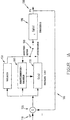•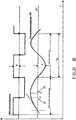•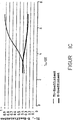•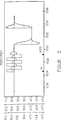•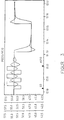•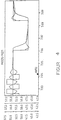•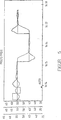•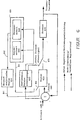•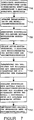•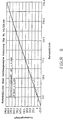•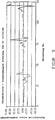•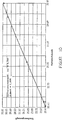•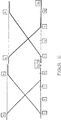## Classifications

• GPHYSICS
• G05CONTROLLING; REGULATING
• G05BCONTROL OR REGULATING SYSTEMS IN GENERAL; FUNCTIONAL ELEMENTS OF SUCH SYSTEMS; MONITORING OR TESTING ARRANGEMENTS FOR SUCH SYSTEMS OR ELEMENTS
• G05B13/00Adaptive control systems, i.e. systems automatically adjusting themselves to have a performance which is optimum according to some preassigned criterion
• G05B13/02Adaptive control systems, i.e. systems automatically adjusting themselves to have a performance which is optimum according to some preassigned criterion electric
• G05B13/0265Adaptive control systems, i.e. systems automatically adjusting themselves to have a performance which is optimum according to some preassigned criterion electric the criterion being a learning criterion
• G05B13/0285Adaptive control systems, i.e. systems automatically adjusting themselves to have a performance which is optimum according to some preassigned criterion electric the criterion being a learning criterion using neural networks and fuzzy logic

## Abstract

A system for tuning a process control loop, the system comprising:
- a voting module (600) for receiving an error signal (680) representative of the difference between a setpoint and a process variable to produce at least a first process control signal for controlling the process (630);
- a non-linear module (660) for generating at least one parameter signal (651);
A controller module (610) for receiving the error signal and the at least one parameter signal from the non-linear module (660), the controller module (610) generating a second process control signal for controlling the process; and
A switching device (670) coupled to the process for coupling either the tuning module (600) or the regulator module (610) to the process to select the first or second process control signal to control the process,
characterized in that
the tuning module (600) uses the error signal (680) to generate a set of characteristic signals of the at least one limit gain Ku, limit period Tu, dead time L ...

## Description

• BACKGROUND OF THE INVENTION
• Scope of the invention
• The present invention relates to a system and method for tuning a process controller based on non-linear estimators of voting rules including neural networks and fuzzy logic.
• Description of the Prior Art
• A proportional, integral, derivative (PID) controller is a controller commonly used in industrial processes, including computer-controlled industrial processes. Such PID controllers and their variations and combinations, for example P, PI, PD, are widely used in the control of industrial processes. Typical industrial processes are governed by one or more control loops with PID controllers.
• A Fuzzy Logic Controller (FLC) is also a well known process controller used to control process parameters by keeping process variables within parameters related to desired setpoints. FLCs are not linear regulators and are increasingly used in industrial environments.
• One type of known method for tuning parameters of a PID controller is the Ziegler-Nichols method. The automatic tuning based on vibrations excited by a relay is also a well-known and accepted technique of automatic tuning. The automatic relay vibration tuning determines the limit gain (Ultimate Gain) and the limit period of a process at the stability limit (Ultimate Period). The settings of the PID controller can be determined from these parameters using the rules of Ziegler-Nichols and modifications thereof. A continuation of the relay vibration tuning beyond the determination of the limit gain and limit period is described in "System and Method for Automatically Tuning a Process Controller", U.S. Patent No. 5,453,925 , granted in September 1995 to Wilhelm K. Wojsznis and Terrance L. Blevins (hereinafter Wojsznis). It is known that the Ziegler-Nichols method has several disadvantages (see HANG CC, ASTRÖM, KJ, HO, WK: Refinements of the Ziegler-Nichols tuning formula. In: IEE Proceedings-D, Vol. 2, 1991, pp. 111-118).
• Significant progress has been made in recent years in the field of model-based tuning, in particular with Internal Model Control (IMC) and Lambda tuning. Both approaches result in a closed loop response of the first order corresponding to the changes in the setpoints. An answer speed related tuning parameter is used to set the trade-off between performance and robustness. Both methods adjust the integral time parameter of the PID controller (or the integral time parameter and the derivative time parameter) to eliminate the pole (s) of the process and adjust the controller gain to achieve the desired closed loop response. IMC and lambda tuning have prevailed as oscillations and oversteer are avoided and the control behavior can be preset in an intuitive way via the closed-loop time constant.
• One of the limitations of model-based tuning is the need to determine a process model. An equivalent first order process plus dead time with the parameters static gain, apparent dead time and apparent time constant is normally determined for self-regulating processes. For processes with integrating behavior, model parameters of the integral gain of the process and the dead time are determined. The determination of the model is typically done by means of a jump test of the open loop. Compared to the method of oscillation excited by a relay, open loop methods are not easily automated. In open-loop methods, non-linearities of the process, valve hysteresis, and load disturbances often require human intervention to ensure the accuracy of a model. Self-regulating and integrating behavior processes require a different technique.
• From an article by Swinarzky (SWINIARSKI, RW: Novel Neural Network-based Self-Tuning PID Controller Which Uses Pattern Recognition Technique., IN: American Control Conference, 1990, pp. 3023- 3024), it is known to use a neural network to generate parameters for the Ziegler-Nichols method. Furthermore, it is apparent from this document that one can use an artificial neural network, in particular a switched neural network, to calculate the parameters for a PID controller. Here, a process is monitored over a longer period of time and derived corresponding parameters for the PID controller. This process is very complicated.
• What is needed is a system and method for tuning in a relay vibration environment that provide the required PID tuning parameters across all ranges of model parameters and determine model parameters of a process.
• SUMMARY OF THE INVENTION
• This object is achieved by a system according to claim 1. Further advantageous embodiments will become apparent from the dependent claims.
• The provided system uses non-linear approaches in the non-linear module to approximate the desired tuning parameters of the controller. The non-linear approaches include neural network tuning, fuzzy logic tuning and non-linear tuning, including sigmoid tuning.
• One system also provides that the non-linear module uses nonlinear approaches to approximate the desired parameters of the process model. According to an embodiment of the present invention, the determination of a process model is achieved by means of neural networks, fuzzy logic and non-linear functions including sigmoid tuning, which can advantageously achieve better model parameters than the state-of-the-art analytical formulas for the determination Base of vibrations excited by a relay.
• BRIEF DESCRIPTION OF THE DRAWINGS
• The present invention and its numerous objects, features and advantages will become more apparent to those skilled in the art from the description of the accompanying drawings.
• 1A FIG. 10 is a schematic block diagram of a relay vibration based voting system according to the present invention. FIG.
• 1B Fig. 10 is a diagram of the tuning according to an embodiment of the present invention.
• 1C Figure 12 is a graphical representation of the non-linear coefficient functions for calculating the integral time and gain of the controller.
• 2 is a plot of the vote and step responses for the process with L / T ≈ 0.2 and loop sampling = 0.1 s.
• 3 is a graphical representation of the tuning and step responses for the process with L / T ≈ 0.5 and control loop sampling = 0.1 s.
• 4 is a plot of the vote and step responses for the process with L / T ≈ 0.7 and loop sampling = 0.1 s.
• 5 is a plot of the vote and step responses for the process with L / T ≈ 0.7, loop sampling = 0.1 s with fast response.
• 6 Figure 13 is a diagram of a neural network assisted voting device.
• 7 FIG. 10 is a flow chart of the neural network development steps required according to an embodiment of the present invention. FIG.
• 8th FIG. 12 is a graphical representation of the neural network predicted results of integral time-actual / required integral time using the method according to an embodiment of the present invention. FIG.
• 9 FIG. 12 is a graphical representation of the results: integral time predicted by the neural network model - actual integral time / required integral time using the method according to an embodiment of the present invention.
• 10 Figure 4 is a graphical representation of the results predicted by the neural network model for the subrange of the T i values 15 to 33 according to an embodiment of the present invention.
• 11 FIG. 12 is a graphical representation of the membership functions of the inputs in an embodiment of the present invention. FIG.
• By using identical reference numerals in different drawings, similar or identical elements are identified.
• DESCRIPTION OF THE PREFERRED EMBODIMENT (S)
• Now be on 1A which is a schematic block diagram of a voting system based on vibrations excited by a relay 100 which can be fixed according to the new non-linear approaches. A process 190 can be any controllable process. in as process variable (PV) represented output signal 130 is from the process 190 provided and in a summer 120 added, in which the PV with a setpoint (S2) 110 is compared. The difference between PV and SP, which determines the tuning system based on relay vibrations 100 is used, the limit gain K u supplies and the intrinsic period T u for a process. As shown, the voting system contains based on relay vibrations 100 a summer 120 , a relay 150 and voting rules 160 , The setpoint (SP) 110 is used together with the process variable (PV) 130 to the summer 120 delivered. The summer 120 subtracts PV from SP and delivers the result to the relay 150 , the voting rules 160 and a regulator 170 , The process is tuned either automatically or under the control of a user. Optionally, the vote of the process 190 include an automatically controlled voting procedure based on the natural vibration, such as the procedure described in Wojsznis. The natural vibration procedure involves a switch 180 to control that either the output of the regulator 170 with the process 190 or the output of the relay 150 with the process 190 is coupled. In one embodiment, the relay performs 150 the natural vibration procedure with the voting rules 160 off by the switch 180 controls. The desk 180 either couples the controller 140 or the voting rules 160 and the relay 150 with the process 190 , The procedure is a vibration procedure that determines the time delay, the limit gain, and the limit period.
• The apparent dead time Td is determined upon initialization of the tuning. The apparent dead time Td is determined by a tangent as the slope of the process output PV 130 during the initialization of the vote is applied. The tangent is extrapolated to intersect the setpoint (SP) or median line of the process output before tuning. The time between the first relay jump and this cut is the apparent dead time. Other methods of determining Td are also within the scope of the present invention. Such other methods include those provided by Wojsznis.
• 1B shows a graphical representation of the process input and output signals during parts of the vibration procedure. The time duration between the time t1 ( 10 ), Switching the relay 150 , and time t2 ( 20 ), at which the process output reaches the maximum, corresponds approximately to the dead time. Otherwise, the dead time is calculated as the difference between the time of the | SP-PV | increment and the time of the | SP-PV | -decrement. The duration of the evaluation in this approach corresponds to the duration of one or more boundary periods (Tu). The apparent dead time can be calculated as an average of two or three results.
• Dead time, limit gain, and limit period are sufficient to calculate a first order process plus dead time. Equations (1) and (2) for calculating the first order plus dead time are: in which:
• T c
= Time constant of the process
T u
= Boundary period
T d
= apparent dead time of the process
K s
= static amplification of the process
K u
= Limit gain.
• The time constant of the process in equation (1) is expressed by a tangent function which gives a good approximation to the arguments smaller than π / 3 when the dead time is relatively large compared to the time constant. For processes with negligible dead time, the calculation of the time constant results in a large error, even with a small error in the specification of the dead time (the tangent argument is approximately equal to π / 2, and a small error in the argument leads to a large error of the tangent value) , Some improvement results from the use of a linear function as shown in equation (3) for arguments greater than π / 3:
• Ziegler-Nichols (ZN) rules for tuning a PID controller
• The tuning based on vibrations excited by a relay naturally conforms to the ZN rules and provides the threshold gain K u and the threshold period T u . The original ZN formulas for PI controllers are as follows: K = 0.4 K u ; and T i = 0.8 T u.
• These formulas yield a phase margin that varies widely between about 20 ° and 90 °, depending on the ratio τ of the dead time L of the process to the time constant T of the process. Consequently, the behavior also varies considerably, from an extremely oscillatory response in a process with a ratio close to 0.1 to an extremely sluggish response in a process with a ratio near 1.0.
• A PID controller that works with the original ZN rules K = 0.6 K u ; T i = 0.5 T u and T d = 0.125 T u was matched, shows a similar behavior. To overcome this problem, various modifications of the original formula have been proposed. A tendency is to reduce the gain and shorten the integral time, as shown by the following formulas: K = 0.4 K u ; T i = 1/3 T u and T d = 1/12 T u .
• The above modification improves the behavior of control loops with τ close to 0.5; However, control loops with small values of τ become even more vibrating.
• Other, more flexible formulas (4), (5), and (6) provide the following for the phase / gain reserve design: T d = alphat i (5) K = K u cosφ / G m (6) in which:
• α
Draft selection of the ratio T d : T i with the default value 0.15.
G m
desired gain reserve with default 2.0.
φ
Phase margin.
K, T d and T i
are the parameters of the controller.
• With a given phase and gain margin, formula (7) provides constant coefficients to compute T i , T d and K from T u and K u . A typical design with φ = 45 ° gives the following coefficients: K = 0.38 K u ; T i = 1.2 T u and T d = 0.18 T u (7)
• This design is suitable for small τ, but gives an extremely sluggish response for τ greater than 0.2.
• If it is assumed that the phase reserve φ = 33 ° and the gain reserve = 3.0, then the following coefficients result in formula (8): K = 0.27 K u ; T i = 0.87 T u and T d = 0.13 T u ; (8th)
• These coefficients are suitable for designing a narrow τ region near 0.25.
• Another known modification sees the definition of controller parameters as functions of the normalized dead time L / L + T or the normalized gain k = 1 / K p K u , where K p is the static gain of the process. However, the above approach uses both the dead time and the time constant or the static gain of the process. It therefore can not be used directly for tuning based on relay vibrations.
• Non-linear estimator of voting rules
• When developing non-linear estimators of voting rules, certain assumptions and considerations should be considered. First, all input parameters are obtained during the relay oscillation test (i.e., the threshold gain, the threshold period, and the dead time). Second, the major shortcoming of the Ziegler-Nichols rule is an unadjusted integral time of the controller in processes of low dead time and excessive integral time in processes of significant dead time. Third, the voting rules should provide controller tuning parameters and responses that are close to those of model-based voting (IMC or lambda).
• The in 1A and 1B illustrated and discussed in the appropriate discussion relay vibration test for the vote provides the limit gain K u and the boundary period T u . To overcome the shortcomings of the ZN rules, non-linear estimators have proven to be an improvement in the definition of voting parameters.
• A sigmoid expression provides a smooth transition between two different values and serves to develop non-linear estimators. The following formulas meet the above requirements: T i = f 1 (T u , L) T u ; K = f 2 (T u, L) K u; T d = D1T i; (9) f 2 = (T u , L) = a 2 + (b 2 -f 1 (T u , L) / c 2 ; (11) a1, a2, b1, b2, c1, c2 are heuristic coefficients and
a1 is between 0.3 and 0.4;
b1 ≈ 0.6; b2 ≈ 1.0; c1 ≈ 7.0; c2 ≈ 4.0; d1 ≈ 0.125; d2 ≈ 1.0.
• Formula (10) gives the value of the coefficient used to calculate T i , which varies between a minimum value of a1 and a maximum value a1 + b1, as in FIG 1C shown. Formula (11) provides the adaptation of the coefficient for the calculation of K in the range: [a2 + (b2 - a1 - b1), a2 + (b2 - a1)].
• This approach results in significantly improved voting responses that approximate those of IMC or lambda tuning (unlike the decay of quarter-wave amplitudes). Some typical step responses for different L / T are in 2 to 5 shown. An example of a closed loop tuning and a step response for a second order process with Gain = 1; T1 = 10 s; T2 = 3 s; L = 2 s is in 2 shown.
• The voting system based on relay vibrations 100 generated the following controller setting: K = 1.65; T i = 12.36 and T d = 1.97 compared to the IMC calculations: K = 1.0; T i = 12.5 and T d = 1.97. 3 shows a graph with L increased up to 5 s, and 4 shows a graph with L increased up to 8 s. As well in 3 as well as in 4 For example, the step responses are similar to the model-based voting, although the integrating behavior is somewhat weaker than required for the IMC response (the tuner gave T i = 15.7 and 18.37 compared to 14.0 and 15.5 according to the IMC calculations).
• A design of the tuner according to the new non-linear approaches of the present invention allows a user to adjust the tuning behavior by providing the choices of slow, normal, and fast. 5 shows z. For example, the step response of the control loop for the process L / T ≈ 0.7 with the option 'Fast'. Using speed selection increases the overall flexibility of the design by better tailoring the tuning response to specific conditions and requirements.
• Now be on 6 in which a voting device based on relay vibrations is shown in a schematic block diagram which optionally applies the novel non-linear approaches according to an embodiment of the invention. The illustrated closed loop system may include both non-linear tuning techniques and nonlinear process modeling techniques. Optionally, the system may include linear tuning techniques and non-linear process modeling techniques or non-linear tuning and linear process modeling techniques.
• As the embodiment according to 6 shows, becomes a process 630 via a switch 670 either with a voting device based on relay vibrations 600 or a controller 610 coupled. The regulator 610 is via a second switch 690 coupled so that it either a first parameter signal 651 from the regulator design 650 or a second parameter signal 641 that from the analytical regulator design 620 and the process model 640 is issued, receives. The regulator 610 provides an output when it is switchable with the process 630 is coupled. The tuning device based on relay vibrations 600 , the process model 640 , the regulator design 650 , the regulator 610 , the process 630 and the analytical 15151515 regulator design 620 can all be implemented as software modules, hardware, or a combination of both.
• As will be described later, the switch is 670 set to the appropriate process control signal to the process 630 to regulate. The tuning device based on relay vibrations 600 is also coupled to send signals to a non-linear module 660 that delivers the process model 640 and the regulator design 650 contains. Both the controller design 650 as well as the process model 640 use neural networks, fuzzy logic, non-linear functions, or other nonlinear or linear techniques, as described below. The tuning device based on relay vibrations 600 and the regulator 610 receive an error signal 680 representative of the difference between a setpoint and a process variable, and generate a first process control signal to control the process 630 , The tuning device based on relay vibrations 600 also provides signals to the non-linear module 660 ,
• The first parameter signal 651 and the second parameter signal 641 be through a switch 690 with the regulator 610 coupled. The output of the process model 640 is with the analytical controller model 620 shown coupled. A parameter signal 641 Contains process model parameters contained in a parameter signal 642 contained by means of non-linear techniques in the process model module 640 is calculated. In a preferred embodiment, the parameter signal includes 641 Furthermore, controller parameters used in the analytical controller design 620 by means of the in the process model module 640 certain and in the signal 642 calculated process model parameters are calculated. One of ordinary skill in the art will appreciate that controller parameters can be generated from process model parameters. Accordingly, the analytical controller design applies 620 optional linear techniques for determining the controller parameters.
• The from the regulator design 650 output parameter signal 651 contains controller parameters that are calculated using non-linear techniques. The one with the regulator 610 coupled switches 690 provides the option to (1) choose the controller parameters that are used with non-linear techniques in the process model 640 generated process model parameters in the analytical controller design 620 be calculated; or (2) to select the controller parameters that are generated by non-linear techniques in the controller design 650 be calculated.
• Alternatively, the analytical controller design is 620 removed from the system implementation in one embodiment. Without the analytical regulator design 620 can the switch 690 both with the parameter signal 651 as well as with the parameter signal 642 be coupled. Accordingly, the switch 690 not required in this embodiment. The regulator 610 So receives both the controller parameters in the parameter signal 651 as well as the process model parameters in the parameter signal 642 , Both parameter signals 651 and 642 become in the non-linear module 660 by non-linear techniques such as neural networks, fuzzy logic, non-linear functional approaches, or other non-linear or linear techniques described below.
• Neural network based voting
• With reference to 6 , Regulator design 650 , the controller parameters are optionally determined by means of a neural network modeling approach. Such a neural network modeling approach improves adaptation of the three PID controller parameters over a wide range of model parameter changes. Control concepts using neural networks are generally divided into two broad categories. One approach involves replacing a regulator with a neural network. The neural network is trained by mimicking a controller or a human expert. In this approach, each loop must be trained separately so that it is not a good candidate for a voting model that requires voting results in a simple and standardized voting procedure. An alternative approach uses neural networks as support in modeling, implementing rule laws, or monitoring. A specific design of a voting device is in 6 shown. The tuner selectively uses neural networks or other non-linear techniques described below to compute the process model and parameters of the PID controller.
• Similar to the natural vibration procedure associated with 1 described uses the voting device of 6 also a natural vibration procedure. The natural vibration procedure provides control of the switch 670 in the way that either the output of the regulator 610 with the process 630 or the output of the tuner based on relay vibrations 600 with the process 630 is coupled. In one embodiment, the controller receives 610 over the switch 690 the controller parameters either from the controller model 650 or from the analytical controller model 620 , In this embodiment, the analytical controller design is obtained 620 Parameter for determining the process model from the process model 640 , In another embodiment, the analytical controller design is 620 taken out of the process control loop. The desk 670 either couples the controller 610 or the voting means based on relay vibrations 600 with the process 630 , The regulator design 650 uses non-linear techniques to determine the controller parameters. A technique described below uses neural networks.
• The procedure for developing neural network techniques involves some basic steps that are described in US Pat 7 are shown. 7 is a flow chart of the steps in creating neural networks. step 700 shows the first step in which simulated loop configurations are developed with the inclusion of future applications of the tuner. Normally a process module of either second or third order plus dead time is used. step 710 gives an assumed area for the changes of the process parameters. step 720 indicates that the designer computes the PID controller settings for each set of model parameters using IMC rules, lambda tuning, or another preferred controller scheme. step 730 provides that the developer can run the auto-tuner for the same amounts of model parameters and the results of voting, T u, u K and L records. step 740 provides that the developer checks the behavior of the loop with the calculated parameters and adjusts the parameters as needed. step 750 For example, the developer trains the neural network using the simulated voting results as inputs to the neural network and the model and controller parameters as outputs of the neural network. step 760 For example, the developer implements the trained neural network in the voting engine.
• The above with reference to 6 described neural network can be of any type (sigmoid or radial basis functions). In one embodiment of the present invention, the neural network is used with the neuron transfer function given by: as neuronal output. In is a weighted sum of external inputs. In = Σw i In i .
• The neural network is selectively applied with multiple outputs (outputs K and T i for the neural network of the controller and K p and T for the neural network of the model) or as multiple neural networks with a single output. The benefit of the single-output neural network is faster training. For this reason, one embodiment implements a neural network with a single output. In an exemplary concept for a voting device, the following inputs and outputs of the neural network are defined:

Inputs: T u , K u , L, noise level, relay hysteresis and sampling speed.
• T u, u K, L, and the noise level are defined during the tuning test.
• The relay hysteresis and the sampling rate are parameters of the tuner.

Outputs: K, T i , T d , K p and T.
• K, T i and T d are parameters of the PID controller.
• K p and T together with L are parameters of the first order process model plus dead time.
• Questions of implementation and test results
• In one example of the new non-linear techniques, neural network models are implemented to enhance the autotuner based on relay-excited oscillations in a scalable controller system for industrial purposes. The autotuner has two blocks: the tuner function block implemented in the controller and the tuner application. The application of the voting device is implemented in a suitable console. The neural network models are added to the voting device application. In one embodiment, the neural network models are transparent to the user of the tuner, the autotuner having no choices or settings associated with neural networks.
• According to the method described here, the neural network is trained for the second order process model plus dead time. The following specification defines the available input and output data for training neural network models:
• Process model based on a neural network
• Inputs: limit gain K u , limit period T u and dead time L, defined during the relay-based tuning experiment.
• Outputs: process gain: 0.5; 1.0; 1.5; Time constant 1 of the process in seconds: 1.0; 2.0; 5.0; 10.0; 20.0; 50.0; 100.0; 200.0; Time constant 2 of the process in seconds: 1.0; 2.0; 5.0; 10.0; Dead time in seconds: 1.0; 2.0; 5.0; 10.0; 20.0; 50.0; 100.0; 200.0.
• Controller model based on a neural network
• Inputs: as for the process model based on a neural network.
• Outputs: gain K of the PID controller, integral time T i and derivative time T d , calculated from the process model parameters given above for the outputs, ie process gain 0.5; 1.0; 1.5; Time constant 1 of the process in seconds: 1.0; 2.0; 5.0; 10.0; 20.0; 50.0; 100.0; 200.0; Time constant 2 of the process in seconds: 1.0; 2.0; 5.0; 10.0; Dead time in seconds: 1.0; 2.0; 5.0; 10.0; 20.0; 50.0; 100.0; 200.0.
• Experimental testing
• An experimental trial of voting simulations has been run 142 times, which is about the minimum number of scans suitable for training a simple neural network. The samples gave a good correlation coefficient and, in general, predicted output values approaching that of the required output. The graphs of the results for T i are in 8th and 9 shown.
• At some intervals of the predicted parameter range, the prediction error was unacceptable. There are two specific cases in this regard. In one case, these are small values of the predicted controller parameters (relative to the prediction error). In this case, even a predicted parameter of only 1% of the maximum value gives unacceptable parameters. As in particular from 8th T i can be zero or even negative if the actual value is close to the prediction error.
• Another error situation occurs when there are too few samples in the subrange. For example, a user would like to extend the field of neural network modeling by looking for an extended sub-range, e.g. B. T i > 50.0 in 8th If only a few simulations are performed, an error could occur.
• Neural network based voting rules
• To avoid anomalies, the neural network tuning models employ the following rules, in accordance with the example non-linear techniques. First, as many samples as possible should be captured. At a minimum, it is necessary to capture the minimum number of samples required for a simple model. Second, the range for the predicted parameter should be chosen such that an in-range parameter changes only a few times (about 5). If the predicted values change more frequently (about 15 times), the range of parameter changes has to be divided into several subregions, and several neural network models have to be developed. Third, small predicted values should be given special attention. If the predicted values are small compared to the prediction error, a separate neural network model has to be created for the small values.
• For example, a model with a narrowed subrange of 15 to 33 (instead of 1 to 320 of the full range) has been developed. Although the number of scans was below the minimum number, the prediction was much better than the original model for the entire range. A graph of the prediction of the neural network model for the subregion of T i is in 10 shown. The prediction error relative to the IMC parameters is less than 5%, while the error of the non-linear function estimator for the test cases L / T> 0.5 exceeds 12.0%.
• Linear and non-linear correction functions for determining the process model
• Now be up again 6 which, together with Formulas (1) and (3) (hereafter repeated), describes the use of linear and non-linear correction functions to indicate the process model. As described above, the process model provides 640 Parameters of the process model by using either linear functions or linear correction functions. The ones described below Techniques apply linear, non-linear functions, neural networks, and fuzzy logic as correction functions to determine the process model that is in the process model 640 be executed.
• Consisting of formulas (1) and (3) generated time constant (T c) is considerably smaller for smaller ratios apparent deadtime of the process to limit gain and an excessively high time constant (T c ) in the apparent dead time ratio of the boundary enhancement process greater than 0.25, as shown in Table 1.
• A correction function f (T u / T d ) applied to the specified time constant of the process T c (corr.) = T c (given) f (T u / T d ), should have f (T u / T d ) values greater than 1 for a small T d / T u and less than 1 for T d / T u greater than 0.25.
• A simple linear function with coefficients developed from simulated tests corrects the time constant of the process according to equation (12):
• In a non-linear function, a sigmoidal expression provides a smooth transition between two different values. A sigmoid correction function is well defined if the minimum and maximum values are known. The following formula (13) for estimating the time constants of the process has been developed on the basis of a small amount of simulated data:
• Formula 12 gives the value of the coefficient used for the calculation of T c , which varies between a minimum value of 0.8 and a maximum value of 2.2. After the correction of the time constant, the static amplification of the process is repeated using the formula ( 2), which is repeated below:
• The tuning and calculation results for the Static Gain 1.0 process are summarized in Table 1 and the Static Gain 2.0 in Table 2. In both cases the process is a second order model with T1 = 10 s; T2 = 2 s plus dead time according to column Td. The identifier approximated the model by a first order model plus dead time (Td determined). The presented model parameters are first given (Td, Kp and Tc) and corrected by means of linear and non-linear estimation formulas (Kp and Tc). limit gain Cross period Td model Td identifier Tc identifier Kp identifier Tc corr. linear function Kp corr. linear function Tc corr. sigmoide function Kp Corr. Sigmoide function 7,812 9.55 1 1.24 4,921 0.434 9.374 0.799 10.42 0.887 5.65 13.4 2 2.2 6.77 0.589 9,728 0.826 10.57 0.895 3.7 19.85 4 4.2 9.7 0, 879 10, 65 0.949 8.92 0.809 2.85 25.5 6 6.2 12.28 1.11 10.35 0.960 10.38 0.963 2.08 35.5 10 10.0 14.4 1,319 9.72 0.956 11.9 1.12
Table 1: Results of the specification of the process model with Kp = 1.0 limit gain Cross period Td model Td identifier Tc identifier Kp identifier Tc corr. linear function Kp corr. linear function Tc corr. sigmoide function Kp Corr. Sigmoide function 12.84 9.75 1 1,142 5,064 0.266 10.99 0.55 10,95 0.55 8.78 13.4 2 1,838 6,879 0.384 12.36 0.66 14.34 0.77 6.43 19.9 4 3.53 10.0 0.514 12,99 0.65 11.69 0.59 5.24 25.45 6 5,748 12,388 0,614 11.59 0.57 10.68 0.53 3.96 35.7 10 9,735 15.315 0.725 10.84 0.54 12.70 0.61
Table 2: Results of the specification of the process model with Kp = 0.5
• The application of both correction functions considerably improved the determination of the process model.
• Fuzzy logic-based determination
• A typical fuzzy logic controller is a non-linear controller type. Unique features of a fuzzy logic controller are the operation and development of the controller. A fuzzy rule algorithm is defined by linguistic rules. The controller input parameters are represented by fuzzy sets. The operation of the controller is well represented by the control surface, especially with controllers that use input parameters.
• The procedure for developing fuzzy logic (FL) functions is similar to the procedure for developing a fuzzy logic controller. The following procedure is for determining a FL correction function. The regulation of processes by means of fuzzy logic is generally known (see Kahlert, Jörg: Fuzzy Control for Engineers, ISBN 3-528-05460-3).
• First, it is assumed that the correction function for the time constant of the process model has the following form: Tc (corr.) = Tc [1 + ΔF]; (14) where ΔF is a fuzzy logical correction function. The value of the function corresponds to the incremental output of the fuzzy logic controller. The potential advantage of using a fuzzy logic function over a normal non-linear function is that the fuzzy logic function has a more flexible structure and can easily accommodate two or more arguments.
• Second, the function is to use two arguments, where the ratio Tu / Td is the first and the bounds gain is the second argument.
• It is possible to use many different and different types of membership functions. For ease of implementation, simple triangle membership functions are to be used for the input parameters and singleton functions for the output signals. 11 and Table 3 show details of the structure of a fuzzy logic correction function. With respect to Table 3, P is positive and N is negative. UG or UP / L N P N N P P NS PL
Table 3: Inference rules for the correction function Negative: N = -0.4; negative small: NS = -0,2; positive: P = 0.5; positive large: PL = 1.2
• A defuzzification of the output signal is true for singleton membership functions. TC model Kp model Tc corr. linear function Tc corr. sigmoide function Tc corr. FL Kp corr. linear function Kp corr. sigmoide function Kp Corr. FL ≈ 11 1 9.374 10.42 10.13 0.799 0.887 0.86 ≈ 11 1 9,728 10.57 9.55 0.826 0.895 0.81 ≈ 11 1 10.65 8.92 10.00 0.949 0.809 0.89 ≈ 11 1 10.35 10.38 10.29 0.960 0.963 0.95 ≈ 11 1 9.72 11.9 10.30 0.956 1.12 0.99 ≈ 11 0.5 10.99 10,95 11.14 0.55 0.55 0.56 ≈ 11 0.5 12.36 14.34 13.54 0.66 0.77 0.73 ≈ 11 0.5 12,99 11.69 13.53 0.65 0.59 0.68 ≈ 11 0.5 11.59 10.68 12.38 0.57 0.53 0.61 ≈ 11 0.5 10.84 12.70 12.16 0.54 0.61 0.59
Table 4: Comparison between specified time constant and process gain from various correction functions
• Experimental results of fuzzy logic simulation
• The fuzzy logic correction function yielded time constants and gain estimates comparable to the estimates using linear and non-linear correction functions.
• According to Table 4, a relay vibration based identifier with added correction functions provides a first order model. plus dead time, which can be used for model-based tuning calculations of a PID controller.
• Both non-linear functions and neural network models significantly improve voting rules based on relay-excited oscillations. Adhering to the established principles in the development of neural network tuning, neural network modeling provides a number of advantages. A user may implement arbitrary voting rules (not necessarily lambda or IMC) and apply the same methodology to develop neural network based voting models. A neural network model developed in the simulation can accommodate certain features of the voting design. Sampling speed and noise levels, which influence the design of the controller, can be easily added as input parameters to the prediction.
• Other embodiments
• Although the systems and methods described herein employ a tuner that calculates the cutoff period and limit gain of a process to develop information, any other type of tuner that measures process characteristics may be used, including closed loop tuners and other closed loop tuners , Furthermore, the factors and control parameters determined by the disclosed systems and methods may be input either by a user or automatically.
• Furthermore, the elements referred to in all of the schematic block diagrams herein may be implemented in hardware or implemented in a suitably programmed digital computer or processor programmed with software, either in the form of separate programs or as modules of a common program.

## Claims (9)

1. System for tuning a process control loop, the system comprising: - a voting module ( 600 ) for receiving an error signal ( 680 ) representative of the difference between a setpoint and a process variable, for generating at least a first process control signal for controlling the process ( 630 ) to create; A nonlinear module ( 660 ) for generating at least one parameter signal ( 651 ); A controller module ( 610 ) for receiving the error signal and the at least one parameter signal from the non-linear module ( 660 ), wherein the controller module ( 610 ) generates a second process control signal for controlling the process; and - a switching device ( 670 ), which is coupled with the process to either the voting module ( 600 ) or the controller module ( 610 ) with the process to select the first or second process control signal to control the process, characterized in that the voting module ( 600 ) based on the error signal ( 680 ) generates a set of characteristic signals indicative of the at least one limit gain Ku, limit period duration Tu, dead time L, which characterize the process, and the nonlinear modulus ( 660 ) applies a non-linear procedure to generate the at least one parameter signal by means of at least one neural network and / or fuzzy logic and / or sigmoidal tuning, using the set of characteristic signals as input, the nonlinear module ( 660 ) a non-linear process determination module ( 640 ) and a nonlinear controller design module ( 650 ), wherein an output of the non-linear process determination module ( 640 ) and an output from the non-linear regulator design module ( 650 ) with the controller module ( 610 ) are coupled.
2. System according to claim 1, wherein the controller module ( 610 ) is further coupled such that it receives the at least one parameter signal ( 651 ) from the non-linear regulator design module.
3. The system of claim 1, further comprising: an analytical controller design module ( 620 ), which works with the nonlinear module ( 660 ), the analytical controller design module ( 620 ) is able to supply the regulator module ( 610 ) a plurality of controller parameters based on a non-linear module ( 660 ) to provide received plurality of parameters of the model.
4. System according to claim 1, wherein the controller module ( 610 ) comprises a proportional, integral and differential controller.
5. The system of claim 1, wherein the neural network uses either a sigmoidal function or a radial basis function.
6. A system according to claim 1, wherein the neural network module uses a sigmoid function in which a neuron transfer function is given, where In is a weighted sum of external inputs that has the form In = Σw i In i .
7. System according to claim 1, wherein the non-linear module ( 660 ) calculates the parameter signals using non-linear estimators with non-linear functions.
8. The system of claim 1, wherein the tuning module determines the characteristic signals based on vibrations excited by a relay.
9. The system of claim 1, wherein sigmoid function heuristic coefficients are used to provide parameters including an integral time constant, a gain factor, and a derivative time constant.
DE2000141439 1999-08-23 2000-08-23 Automatic tuning device for a loop based on non-linear estimators of tuning rules Active DE10041439B4 (en)

## Priority Applications (2)

Application Number Priority Date Filing Date Title
US150323 1998-09-09
US15032399P true 1999-08-23 1999-08-23

## Publications (2)

Publication Number Publication Date
DE10041439A1 DE10041439A1 (en) 2001-03-01
DE10041439B4 true DE10041439B4 (en) 2011-08-11

# Family

## Family Applications (1)

Application Number Title Priority Date Filing Date
DE2000141439 Active DE10041439B4 (en) 1999-08-23 2000-08-23 Automatic tuning device for a loop based on non-linear estimators of tuning rules

## Country Status (3)

JP (1) JP3512376B2 (en)
DE (1) DE10041439B4 (en)
GB (1) GB2353608B (en)

## Families Citing this family (3)

* Cited by examiner, † Cited by third party
Publication number Priority date Publication date Assignee Title
CN103293953B (en) * 2008-01-31 2017-10-31 费希尔-罗斯蒙特系统公司 The adaptive model predictive controller of robust with the regulation for compensation model mismatch
CN102331717A (en) * 2011-10-10 2012-01-25 哈尔滨工程大学 Intelligent control method of navigational speed of ship
KR101478450B1 (en) * 2013-07-19 2014-12-31 재단법인대구경북과학기술원 Design method of variable pid gains for multivariable nonlinear systems

## Citations (2)

* Cited by examiner, † Cited by third party
Publication number Priority date Publication date Assignee Title
US5453925A (en) * 1993-05-28 1995-09-26 Fisher Controls International, Inc. System and method for automatically tuning a process controller
DE19748718A1 (en) * 1997-11-05 1999-05-06 Hans Werner Dr Ing Philippsen Self-adjusting device for process control system

## Family Cites Families (3)

* Cited by examiner, † Cited by third party
Publication number Priority date Publication date Assignee Title
SE447608B (en) * 1985-04-03 1986-11-24 Hightech Network Ab Method and apparatus for Adjustment of a digital controller
JPS6266301A (en) * 1985-09-18 1987-03-25 Yamatake Honeywell Co Ltd Auto tuning controller
US5742503A (en) * 1996-03-25 1998-04-21 National Science Council Use of saturation relay feedback in PID controller tuning

## Patent Citations (2)

* Cited by examiner, † Cited by third party
Publication number Priority date Publication date Assignee Title
US5453925A (en) * 1993-05-28 1995-09-26 Fisher Controls International, Inc. System and method for automatically tuning a process controller
DE19748718A1 (en) * 1997-11-05 1999-05-06 Hans Werner Dr Ing Philippsen Self-adjusting device for process control system

## Non-Patent Citations (4)

* Cited by examiner, † Cited by third party
Title
ABE, N., SEKI, K., KANOH, H.: Internal Model Control for Single Tubular Heat Exchanger System. In: IECON '94, 20th International Conference on Industrial Electronics, 1996, S. 1165-1170 *
HANG, C.C., ASTRÖM, K.J., HO, W.K.: Refinements of the Ziegler- Nichols tuning formula. In: IEE Proceedings-D, Vol. 138, Nr. 2, 1991, S. 111-118 *
KAHLERT, J.: Fuzzy-Control für Ingenieure, Vieweg Verlagsgesellschaft, 1995, ISBN 3-528-05460-3 *
SWINIARSKI, R.W.: Novel Neural Network based Self-Tuning PID Controller Which Uses Pattern Recognition Technique. In: American Control Conference, 1990, S. 3023-3024 *

## Also Published As

Publication number Publication date
JP2001100803A (en) 2001-04-13
JP3512376B2 (en) 2004-03-29
GB2353608B (en) 2003-10-08
DE10041439A1 (en) 2001-03-01
GB0020529D0 (en) 2000-10-11
GB2353608A (en) 2001-02-28

## Similar Documents

Publication Publication Date Title
Polycarpou et al. Stable adaptive tracking of uncertain systems using nonlinearly parametrized on-line approximators
Huang et al. Fuzzy model predictive control
Bernstein Nonquadratic cost and nonlinear feedback control
US7317953B2 (en) Adaptive multivariable process controller using model switching and attribute interpolation
EP0907909B1 (en) Nonlinear-approximator-based automatic tuner
US5570282A (en) Multivariable nonlinear process controller
US6381564B1 (en) Method and system for using response-surface methodologies to determine optimal tuning parameters for complex simulators
Leu et al. Robust adaptive fuzzy-neural controllers for uncertain nonlinear systems
US7213006B2 (en) Method and apparatus for training a system model including an integrated sigmoid function
EP0680630B1 (en) Parameterized neurocontrollers
US5859773A (en) Residual activation neural network
Bartoszewicz Discrete-time quasi-sliding-mode control strategies
EP0823078B1 (en) Feedback method for controlling non-linear processes
Loh et al. Adaptation in the presence of a general nonlinear parameterization: An error model approach
He et al. Composite nonlinear control with state and measurement feedback for general multivariable systems with input saturation
Ge et al. Adaptive neural control of nonlinear time-delay systems with unknown virtual control coefficients
CA2118598C (en) A closed loop neural network automatic tuner
Hägglund et al. Revisiting the Ziegler‐Nichols tuning rules for PI control
US5159547A (en) Self-monitoring tuner for feedback controller
US4602326A (en) Pattern-recognizing self-tuning controller
US8280533B2 (en) Continuously scheduled model parameter based adaptive controller
Wojsznis et al. Practical approach to tuning MPC
Hong et al. Practical adaptive neural control of nonlinear systems with unknown time delays
US5691896A (en) Field based process control system with auto-tuning
FI111106B (en) Procedure for setting a process control loop in an industrial process

## Legal Events

Date Code Title Description
8110 Request for examination paragraph 44
R018 Grant decision by examination section/examining division
R020 Patent grant now final

Effective date: 20111112